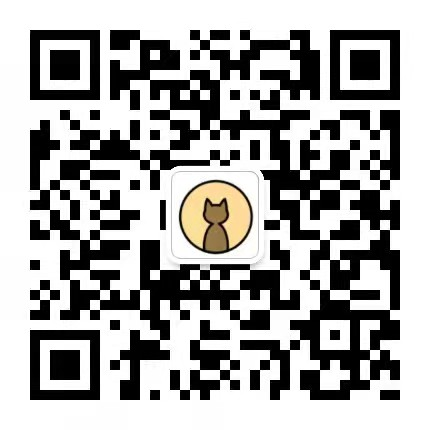2022-05-13

## Sum and Product

Sum and Product

``````Peter: I don't know the numbers.
Sandy: I don't know the numbers.
Peter: I don't know the numbers.
Sandy: I don't know the numbers.
Peter: I don't know the numbers.
Sandy: I don't know the numbers.
Peter: I don't know the numbers.
Sandy: I don't know the numbers.
Peter: I don't know the numbers.
Sandy: I don't know the numbers.
Peter: I don't know the numbers.
Sandy: I don't know the numbers.
Peter: I don't know the numbers.
Sandy: I don't know the numbers.
Peter: I do know the numbers.``````

``````from collections import defaultdict

# build pairs
pairs = []

for i in range(1, 100):
for j in range(i, 100):
pairs.append((i, j))

def singles_operation(f):
results = defaultdict(list)
for a, b in pairs:
results[f(a, b)].append((a, b))

singles = []
for (k, value) in results.items():
# We want to return only the product/sum with one result because if
# peter/sandy have this product/sum, then they'll know the two numbers
if len(value) == 1:
singles.extend(value)

return sorted(singles, key=lambda x: x)

def remove_products():
singles = singles_operation(lambda x, y: x * y)

print('peter removed', singles)
for s in singles:
pairs.remove(s)

def remove_sums():
singles = singles_operation(lambda x, y: x + y)

print('sandy removed', singles)
for s in singles:
pairs.remove(s)

for i in range(7):
remove_products()
remove_sums()

# This is the result because it returns the only pair with a product
#   not created by anything else left in the list.
print('result', singles_operation(lambda x, y: x * y))``````

## 红眼睛、蓝眼睛悖论

1. 他们不能照镜子，不能看自己眼睛的颜色。
2. 他们不能告诉别人对方的眼睛是什么颜色。
3. 一旦有人知道了自己的眼睛颜色，他就必须在当天夜里自杀。